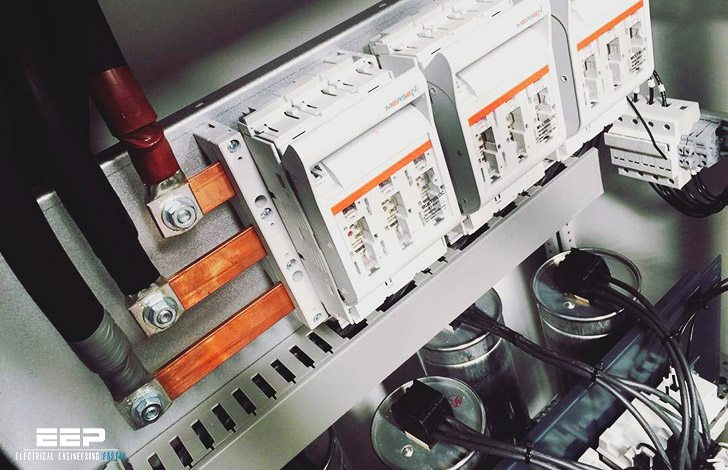Taizhou Dingfeng Electric Appliance Co.,Ltd

## Dingfeng Capacitor--How capacitors improve the power factor and how to calculate them?

Time: 2019-04-19
Summary: It’s quite simple. By installing capacitors or capacitor banks. Improving the power factor of an electrical installation consists of giving it the means to “produce” a certain proportion of the reactive energy it consumes itself.
How capacitors improve the power factor and how to calculate them?It’s quite simple. By installing capacitors or capacitor banks. Improving the power factor of an electrical installation consists of giving it the means to “produce” a certain proportion of the reactive energy it consumes itself.
Power diagram
Power factor is the ratio of working power to apparent power. It measures how effectively electrical power is being used.

Low power factor means you’re not fully utilizing the electrical power you’re paying for. A high power factor signals efficient utilization of electrical power, while a low power factor indicates poor utilization of electrical power.
To determine power factor (PF), divide working power (kW) by apparent power (kVA). In a linear or sinusoidal system, the result is also referred to as the cosine θ.

PF = kW / kVA = cosine θ kVA

For example, if you had a boring mill that was operating at 100 kW and the apparent power consumed was 125 kVA, you would divide 100 by 125 and come up with a power factor of 0.80.

(kW) 100 / (kVA) 125 = (PF ) 0.80

Power diagram
Power diagram

Where:

P – Active power
S1 and S2 – apparent powers
(before and after compensation)
Qc – capacitor reactive power
Q1 – reactive power without capacitor Q2: reactive power with capacitor
Equations:

Q2 = Q1 – Qc
Qc = Q1 – Q2
Qc = P×tg φ1 – P×tgφ2
Qc = P×(tg φ1 – tg φ2)
Where φ1 is phase shift without capacitor and φ2 is phase shift with capacitor

The capacitor is a receiver composed of two conductive parts (electrodes) separated by an insulator. When this receiver is subjected to a sinusoidal voltage, the current and therefore its power (capacitive reactive) is leading the voltage by 90°.

Conversely, for all other receivers (motors, transformers, etc.) the current and therefore its power (reactive inductive) is lagging the voltage by 90°.

The vectorial composition of these currents or reactive powers (inductive and capacitive) gives a resulting current or power below the value which existed before the capacitors were installed.
In simple terms, it is said that inductive receivers (motors, transformers, etc.) consume reactive energy whereas capacitors (capacitive receivers) produce reactive energy.